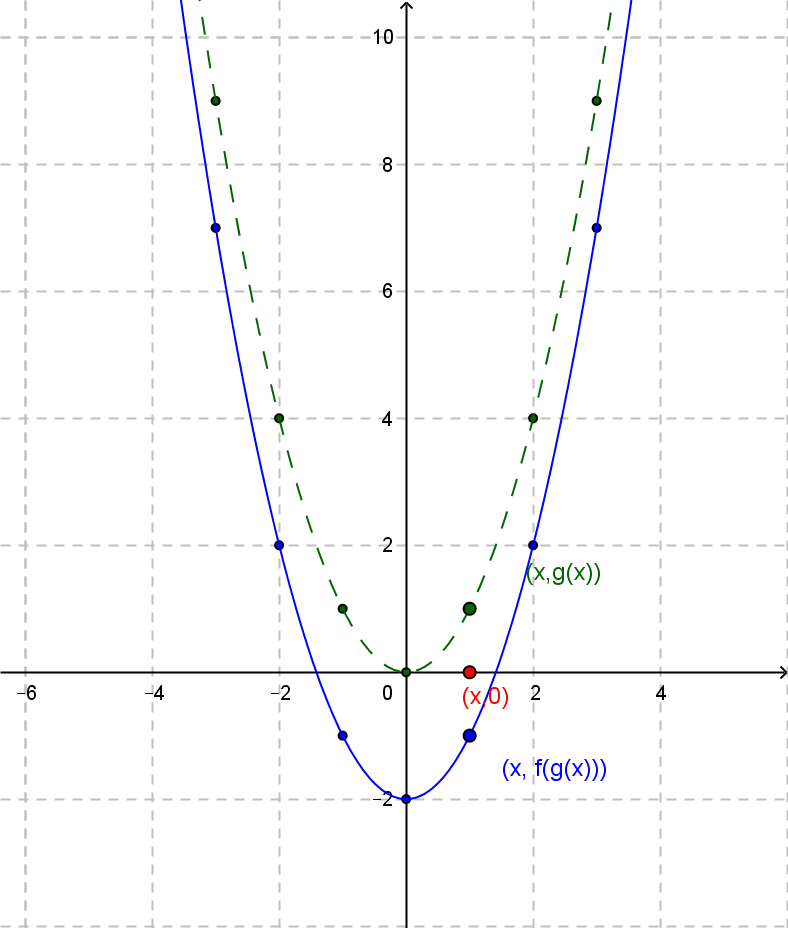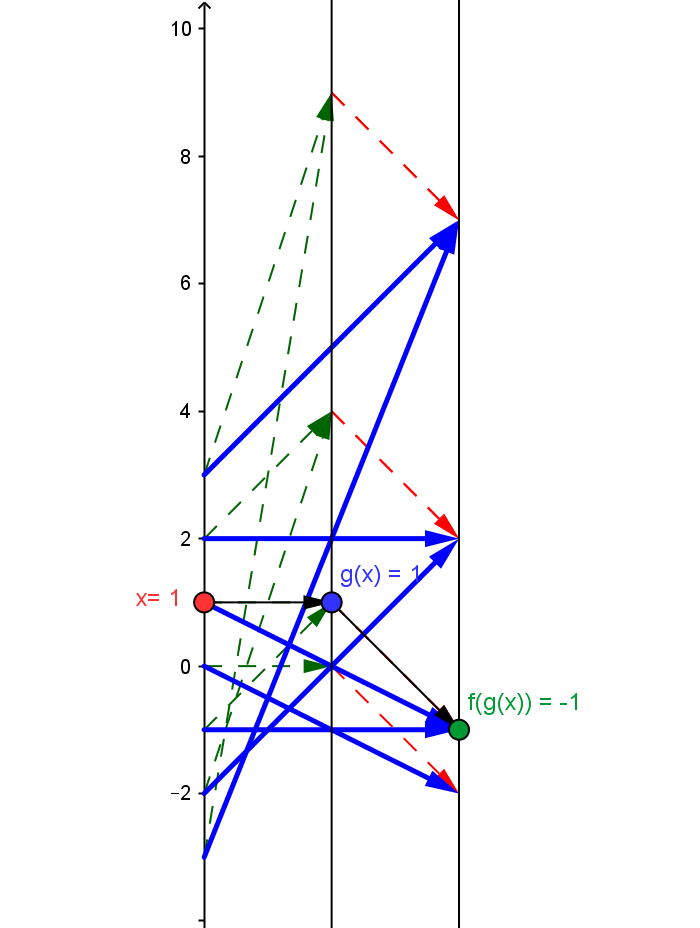Example QF.COMP.2 :\$ f(x) = x - 2; g(x) = x^2\$
The composition of the core quadratic function \$g(x)=x^2\$ followed by the core linear function \$f(x) = x-2\$, so \$q(x) = f(g(x)) = x^2 - 2\$.
Draw a mapping diagram yourself or  use the diagram created with GeoGebra to explore the diagram further.
Compare the mapping diagram with the graphs of \$g(x)\$ and \$f(x)\$
 Graphs of \$g(x)\$ and \$f(x)\$Mapping Diagram Showing Composition. Given a point / number, \$x\$, on the source line, there is a blue arrow  meeting the target line at the point / number, \$ x^2 - 2\$ This point corresponds to the quadratic function's value for \$x\$. The values for the core mapping diagram for \$x-2\$ in red are  applied to the values \$g(x)=x^2\$ (green) .As \$x\$ increases, \$q(x)\$ decreases to value \$q(0)=-2\$ and then increases.
For any \$a \gt 0\$ the even symmetry with respect to \$x=0\$ of \$q\$ gives \$q(+a) = q(-a)\$